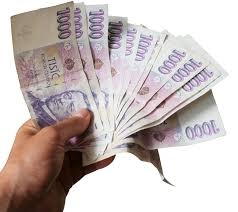# Banknotes 27361

We have the amount of 1,250 euros in banknotes of 100 and 50 euros. We have a total of 17 banknotes. How many are there?

a =  8
b =  9

### Step-by-step explanation:

a+b=17
100a+50b=1250

a+b=17
100·a+50·b=1250

a+b = 17
100a+50b = 1250

Pivot: Row 1 ↔ Row 2
100a+50b = 1250
a+b = 17

Row 2 - 1/100 · Row 1 → Row 2
100a+50b = 1250
0.5b = 4.5

b = 4.5/0.5 = 9
a = 1250-50b/100 = 1250-50 · 9/100 = 8

a = 8
b = 9

Our linear equations calculator calculates it.Did you find an error or inaccuracy? Feel free to write us. Thank you!

Tips for related online calculators
Do you have a system of equations and looking for calculator system of linear equations?